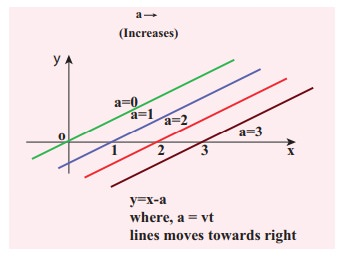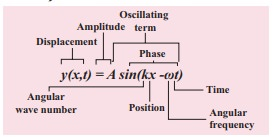Home | | Physics 11th std | Equation of a plane progressive wave (or) Travelling Wave

# Equation of a plane progressive wave (or) Travelling Wave

Let us assume that the wave pulse created during this disturbance moves along positive x direction with constant speed v.

Equation of a plane progressive waveSuppose we give a jerk on a stretched string at time t = 0s. Let us assume that the wave pulse created during this disturbance moves along positive x direction with constant speed v as shown in Figure 11.23 (a). We can represent the shape of the wave pulse, mathematically as y = y(x, 0) = f(x) at time t = 0s. Assume that the shape of the wave pulse remains the same during the propagation. After some time t, the pulse moving towards the right and any point on it can be represented by x' (read it as prime) as shown in Figure 11.23 (b). Then,Similarly, if the wave pulse moves towards left with constant speed v, then y = f(x + vt). Both waves y = f(x + vt) and y = f(x − vt) will satisfy the following one dimensional differential equation known as the wave equationwhere the symbol ∂ represent partial (read ∂y/∂x as partial y by partial x). Not all the solutions satisfying this differential equation can represent waves, because any physical acceptable wave must take finite values for all values of x and t. But if the function represents a wave then it must satisfy the differential equation. Since, in one dimension (one independent variable), the partial derivative with respect to x is the same as total derivative in coordinate x, we writeThis can be extended to more than one dimension (two, three, etc.). Here, for simplicity, we focus only on the one dimensional wave equation.

### EXAMPLE 11.11

Sketch y = x −a for different values of a.

### SolutionThis implies, when increasing the value of a, the line shifts towards right side. For = vt, y = x  vt satisfies the differential equation. Though this function satisfies the differential equation, it is not finite for all values of x and t. Hence, it does not represent a wave.

### EXAMPLE 11.12

How does the wave y = sin(x − a) for a = 0, a = π/4, a = π/2, a = 3π/2, a = π look like?. Sketch this wave.

### SolutionFrom the above picture we observe that y = sin (x−a) for a = 0, a = π/4, a = π/2, a = 3π/2 and a = π, the function y = sin (x−a) shifts towards right. Further, we can take a = vt and v = π/4 ,  and sketching for different times t = 0st = 1 st = 2s etc., we once again observe that y = sin(xvt) moves towards the right. Hence, y = sin(xvt) is a travelling (or progressive) wave moving towards the right. If y = sin(x+vt) then the travelling (or progressive) wave moves towards the left. Thus, any arbitrary function of type = f(xvt) characterising the wave must move towards right and similarly, any arbitrary function of type y = f(x+vt) characterizing the wave must move towards left.

### EXAMPLE 11.13

Check the dimensional of the wave y = sin(xvt). If it is dimensionally wrong, write the above equation in the correct form.

### Solution

Dimensionally it is not correct. we know that y = sin(xvt) must be a dimensionless quantity but xvt has dimension. The correct equation is y = sin (k xωt), where and ω have the dimensions of inverse of length and inverse of time respectively. The sine functions and cosine functions are periodic functions with period 2π. Therefore, the correct expression is y = sin ([2π/λ]x, [2π/T]t) where λ and T are wavelength and time period, respectively.

In general, y(x,t)=A sin(k xωt).Study Material, Lecturing Notes, Assignment, Reference, Wiki description explanation, brief detail
11th Physics : UNIT 11 : Waves : Equation of a plane progressive wave (or) Travelling Wave |